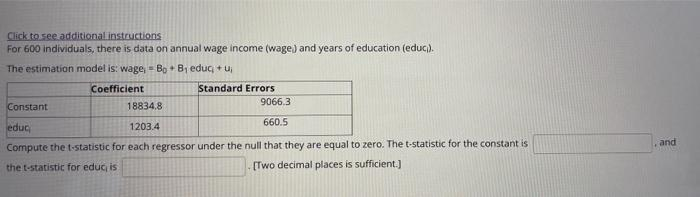# Question Click to see additional instructions For 600 individuals, there is data on annual wage income (wage) and years of education (educi). The estimation model is: wage, Bo + B₁ educ + U₁ Coefficient Standard Errors Constant 18834,8 9066.3 660.5 educ 1203.4 Compute the t-statistic for each regressor under the null that they are equal to zero. The t-statistic for Click to see additional instructions For 600 individuals, there is data on annual wage income (wage) and years of education (educi). The estimation model is: wage, Bo + B₁ educ + U₁ Coefficient Standard Errors Constant 18834,8 9066.3 660.5 educ 1203.4 Compute the t-statistic for each regressor under the null that they are equal to zero. The t-statistic for the constant is the t-statistic for educ, is [Two decimal places is sufficient.] and